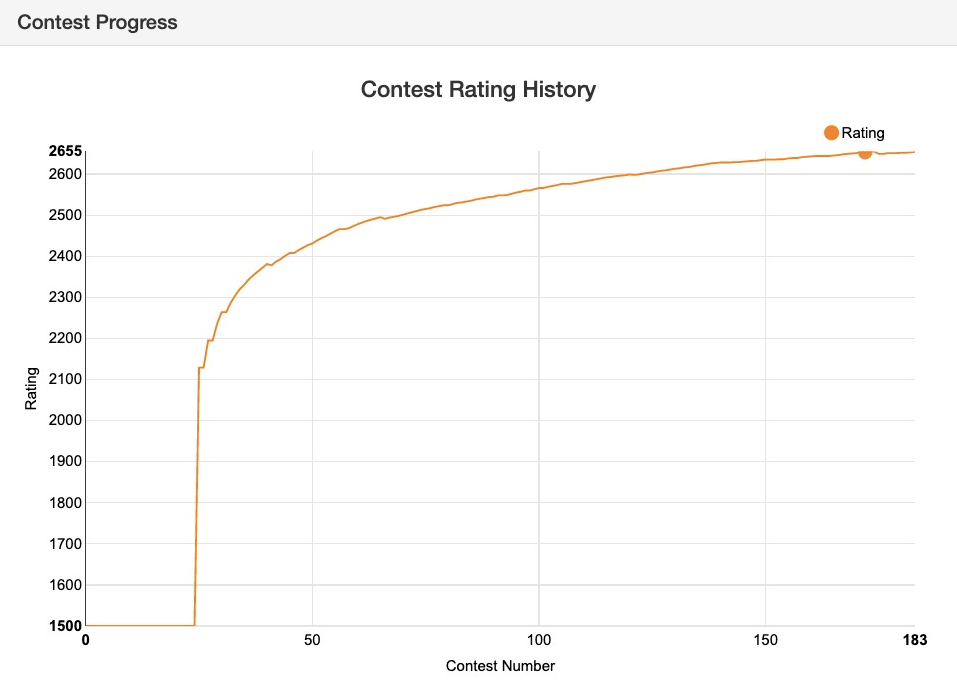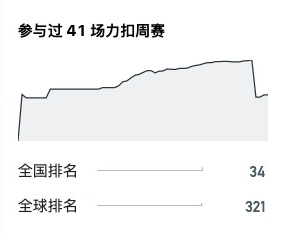📣 全新「周赛评分算法」即将上线，现邀扣友们前来出谋划策！### 0x01 新算法的目标

1. 用户在参加了很多次比赛以后，旧算法的分数更新非常小：1. 旧算法下，用户如果忘记参赛，分数会被扣非常多：1. 在解决问题 1 的同时，分数不能波动太大。

2. 我们会把忘记参赛的人特判出来，不算在参赛者里。但是为了避免 “保分” 的现象，依然需要给忘记参加的用户一些惩罚

### 0x02 ELO 算法介绍

$E_{A, B}=\frac{1}{1+10^{(Rating_B-Rating_A)/400}}$

$Rating^\prime_A=Rating_A+K(S_A-E_{A, B})$

### 0x03 新算法介绍

$ERank_i=\sum^n_{j=1}E_{j, i}+1 (j \neq i)$

$m_i=\sqrt{ERank_i \times Rank_i}$

$\sum^n_{j=1}\frac{1}{1+10^{(Rating_j-ERating_i)/400}}+1=m_i$

$\Delta = f(k) \times (ERating_i - Rating_i)$

$Rating^\prime_i=Rating_i+\Delta$

### 0x06 一些结论

• 从图上可以看出，新的算法可以有效解决分数收敛过快的问题，同时在用户参赛一定场次以后，分数依然会根据排名有可见的波动。

• 经过我们的对比，用新算法得到的前十名的平均竞赛排位比用旧算法得到的更靠前，说明新算法可以更准确地反映选手的算法水平。

• 新算法对最近几场比赛更为看重，在近期的比赛中排名靠前的选手的分数普遍更高。相比旧算法，新算法更具时效性。

👉 戳我

### 0x08 大家来讨论

• 力扣平台非常重视用户的成长。我们观察到有一些用户在一段时间内的水平得到了很大的提升，但由于他们参加了很多场周赛，每次的加分没有真实反映他们的算法水平。所以在这里征询以下大家的意见，我们是否需要赛季？如果需要的话，我们提供两个长度选项：

• 半年

• 一年

• 我们应该如何给忘记参赛的用户惩罚？我们提供两个选项：

• 算分数的时候扣一个固定百分比的分（如 $3%$），e.g. 如果比赛前是 2000 分且忘记参赛了，那么比赛后就是 1940 分。（目前采用的方案）

• 一年（或者一赛季）一次【免死金牌】，忘记参赛也不扣分。第二次及以后的忘记参赛会以输给所有人来计算分数。

26

13

1、可以有赛季，全体评分（即目前的评分）和赛季评分共存。个人认为赛季不宜过长，半年比较合适。
2、忘记参赛的用户惩罚，第一个选项我不赞同，理由是固定百分比，改成固定分数比较合适，因为分数高的用户参赛次数多，一次忘记参赛在所有报名的比赛中所占比例小，如果固定百分比扣分，就是没有考虑到不参赛的次数占报名总次数的比例。第二个选项我比较赞同，一赛季一次免死金牌比较合适，一个小的建议是第二次及以后的忘记参赛按照该次比赛得零分计算分数（每次比赛四道题根据难度高低有各自的分数，正确提交即可得到该题分数，零分意味着没有一道题正确提交，可能是只有错误提交或者没有任何提交，我认为忘记参赛应该和得零分等同，就像高考缺考和参加考试但是全部题都做错的结果一样都是零分）。
3、提一个另外的建议，和周赛评分算法不直接相关，但是可能间接相关。目前的周赛规则为，每次提交只有两种可能，正确提交和错误提交，无论是答案错误还是TLE、MLE都是错误提交，正确提交得全部分，错误提交得零分。为了使比赛更具备区分度，建议考虑根据测试用例的通过率进行评分，通过全部测试用例得全部分，通过部分测试用例得部分分，具体得分和测试用例通过率的关系每道题都可以不一样，但是计算测试用例通过率的时候必须考虑所有的测试用例，而不是只要有一个测试用例不通过就不考虑后面的测试用例，例如一道题有100个测试用例，一次提交的代码没有通过第11个测试用例和第21个测试用例，其余的测试用例都通过，则这次提交的测试用例通过率应为98/100（而不是10/100或19/100）。

11

3

3

1. 赛季问题
建议每一年作为一个赛季。 因为每次秋招也是针对某一年毕业的学生。 可以通过一年的学习来判断自己的实力是否够面试。另外， 如果迟迟不更新会导致分数“通货膨胀”比较严重。（典型例子如cf 2100+不一样）。

2. 忘记参赛。
建议参考cf平台上的， 只要未提交就不算做没有参加。 （但是提交错误一题也没过就算输给所有人了）

@stormsunshine 和他的第三条我有些许不同意见。

easy: n = 2, 1 $\leq m \leq$ 8
hard: 1 $\leq n, m \leq$ 8

$O(n!)$ 8 10
$O(3^n)$ 8 12
$O(2^n)$ 12 24
$O(n^4)$ 50 100
$O(n^3)$ 200 500
$O(n^2logn)$ 782 1536
$O(n^2)$ 2000 1e4
$O(n \sqrt{n})$ 5e4 1e5
$O(nlogn)$ 2e5 5e5
$O(n)$ 1e6 1e7
$O(logn)$ 1e9 1e18

2

2

2

@LeetCode 新排名啥时候更新啊？

1